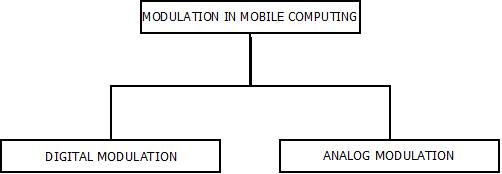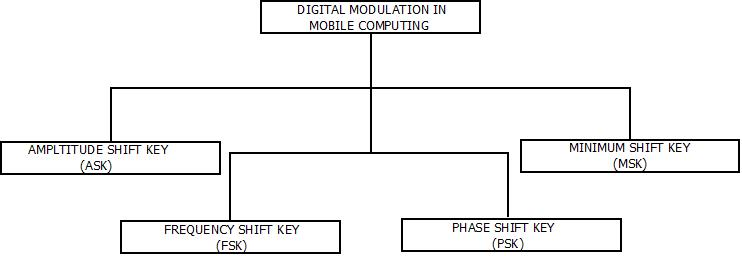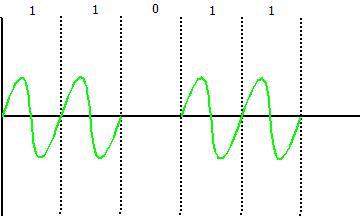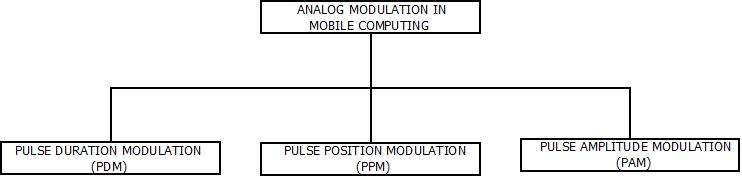# MODULATION MOBILE COMPUTING

Modulation Mobile Computing | Mobile Computing Tutorial | Minigranth

# Modulation : Introduction

• Modulation is the process of converting signals from one form into other form i.e. Analog to Digital or Digital to Analog.

For Example : Consider an Analog transmission medium is available to transmit signals, but we have a digital signal which needs to be transmitted through this Analog medium. This can be done by converting the digital signal into analog signal. This process of conversion is called as modulation.

• This can be classified into two types:Modulation : Mobile Computing

### Modulation : Digital Modulation

• Modulating a signal digitally, is a technique with the help of which digital signals/data can be converted into analog signals i.e. base band signals.
• This can further be classified as:Modulation : Digital

• In Amplitude Shift Key as the name suggests, amplitude is represented by “1” and if the amplitude doesn’t exists, it is represented by “0”.
• Use of Amplitude Shift Key is very simple and requirement of bandwidth is very low.
• ASK is vulnerable to inference or deduction.Amplitude Shift Key

2. Frequency Shift Key(FSK)

• It uses different notations f1 and f2 are used for different frequencies.
• f1 is used to represent bit “1” and f2 is used to represent bit “0”.
• Frequency Shift Key is simple too, but due to use of different frequencies for different bits, bandwidth requirement becomes high.Frequency Shift Key

3. Phase Shift Key(PSK)

• In this, phase difference is used to differentiate between the bits i.e. “1” and “0”.
• If the bit is “1” , simple wave is drawn and if the bit becomes “0”, the phase of the wave is shifted by “180 or π”
• PSK is more complex than ASK and FSK and is robust too.Phase Shift Key

### Modulation : Analog Modulation

• Analog Modulation is a technique with the help of which analog data signals can be transmitted into digital signals i.e. Broadband Signals.
• This can further be classified as :Modulation : Analog